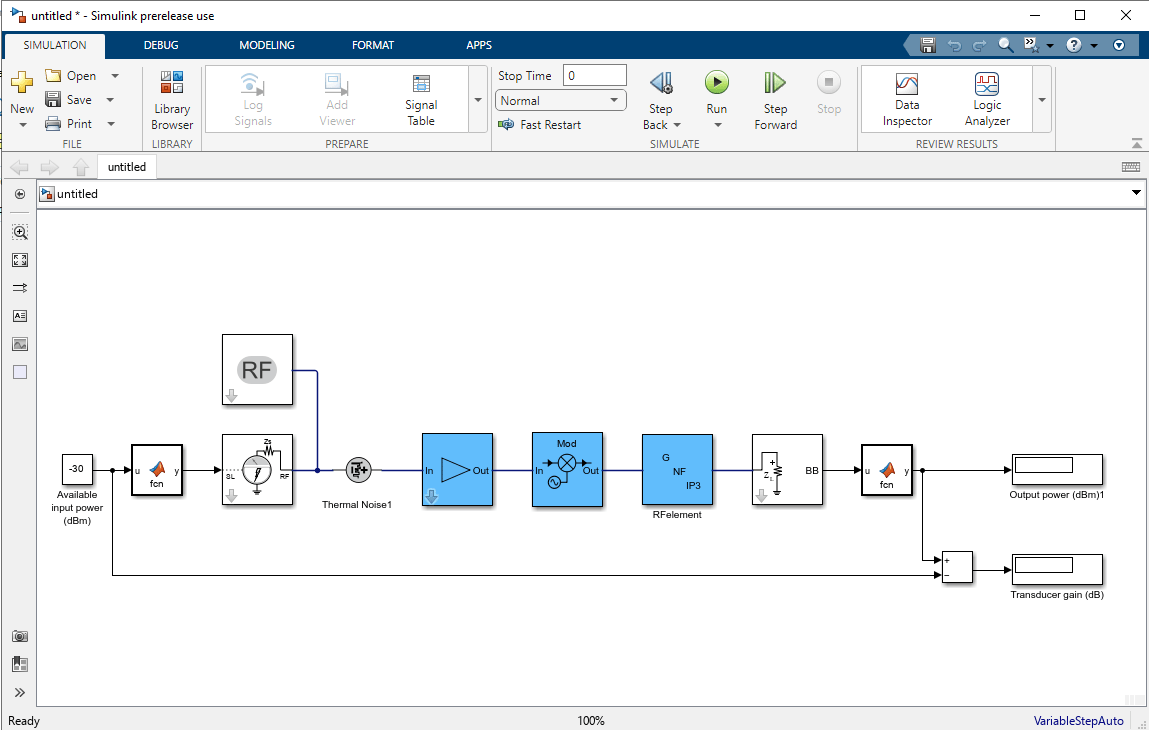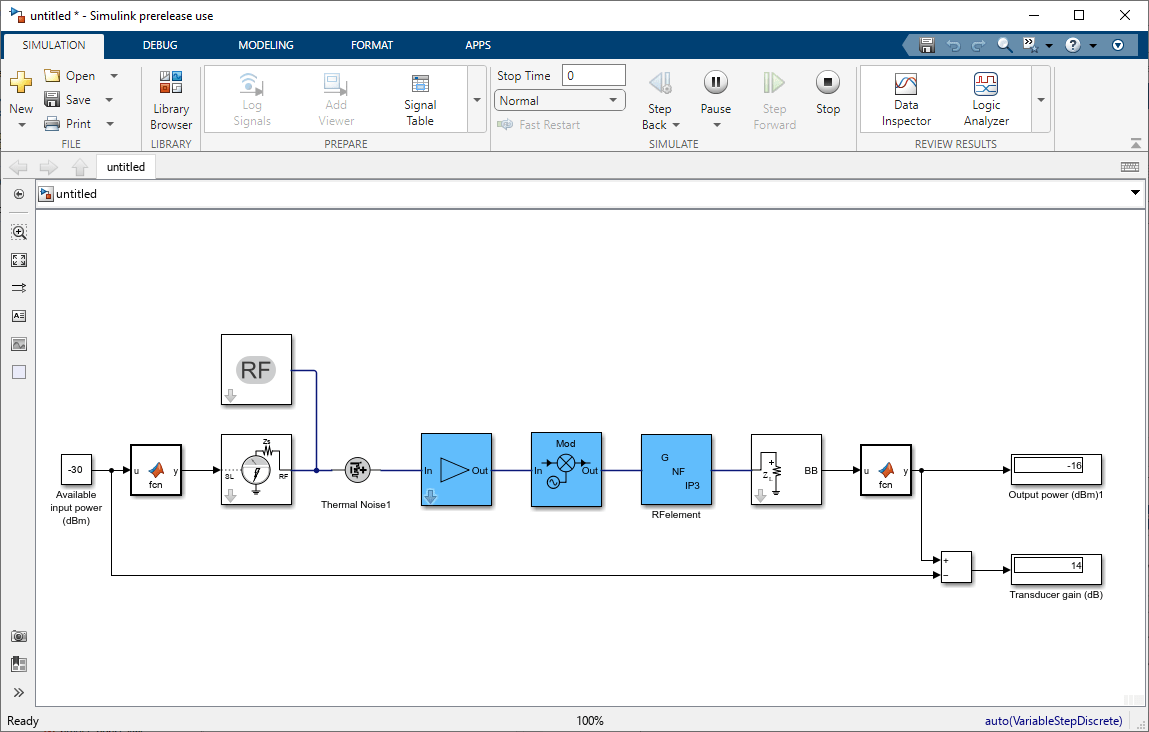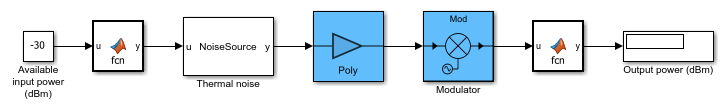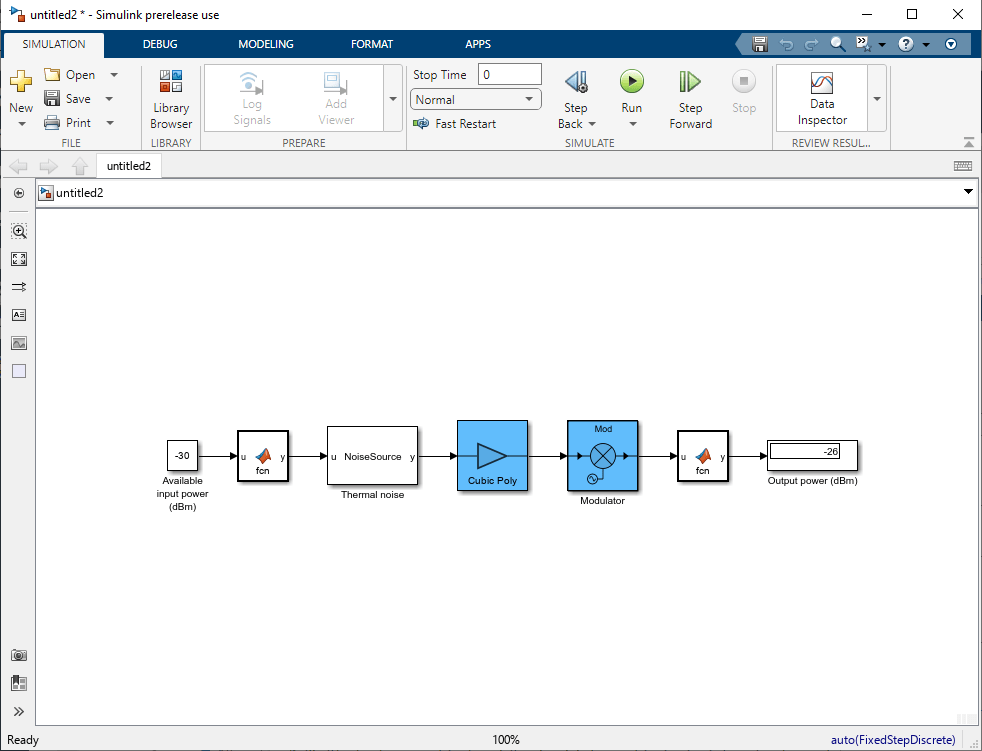# exportRFBlockset

Export RF budget object to RF Blockset Model

## Syntax

``exportRFBlockset(rfobj)``
``exportRFBlockset(rfobj,Library='IdealizedBaseband')``
``sys = exportRFBlockset(___)``

## Description

example

````exportRFBlockset(rfobj)` exports the RF budget object `robj` to an RF Blockset™ Circuit Envelope model.```

example

````exportRFBlockset(rfobj,Library='IdealizedBaseband')` exports the RF budget object to an RF Blockset Idealized Baseband model. (since R2023a)```
````sys = exportRFBlockset(___)` returns the system name. NoteYou need an RF Blockset license to use the `exportRFBlockset` function. ```

## Examples

collapse all

Create an amplifier with a gain of 4 dB.

`a = amplifier(Gain=4);`

Create a modulator with an OIP3 of 13 dBm.

`m = modulator(OIP3=13);`

Create an RF element with a gain of 10 dB.

`r = rfelement(Gain=10);`

Calculate the RF budget of a series of RF elements at an input frequency of 2.1 GHz, an available input power of –30 dBm, and a bandwidth of 10 MHz.

`rfobj = rfbudget([a m r],2.1e9,-30,10e6);`

Create RF Blockset™ model from the RF budget object.

```exportRFBlockset(rfobj) ```Select Run from the Simulate section to run your RF Blockset model.Since R2023a

Simulate a chain of two-port elements in RF Blockset using Idealized Baseband Library blocks to take advantage of the shorter simulation times by assuming perfect impedance matching and a nominal impedance of 1 ohm.

Create an amplifier with a gain of 4 dB.

```a = amplifier(Gain=4); ```

Create a modulator with an OIP3 of 13 dBm.

```m = modulator(OIP3=13); ```

Calculate the RF budget of a series of RF elements at an input frequency of 2.1 GHz, an available input power of – 30 dBm, and a bandwidth of 10 MHz.

```rfobj = rfbudget([a m ],2.1e9,-30,10e6); ```

Export the RF budget object to an RF Blockset model created using Idealized Baseband library blocks.

```exportRFBlockset(rfobj,Library='IdealizedBaseband') ```Click Run on the Simulate tab to run your RF Blockset model.## Input Arguments

collapse all

RF budget object, specified as a `rfbudget` object.

Note

You cannot export an RF budget object to an RF Blockset Idealized Baseband model if one of the elements in the object is an `rfelement`. (since R2023a)

## Version History

Introduced in R2017a

expand all